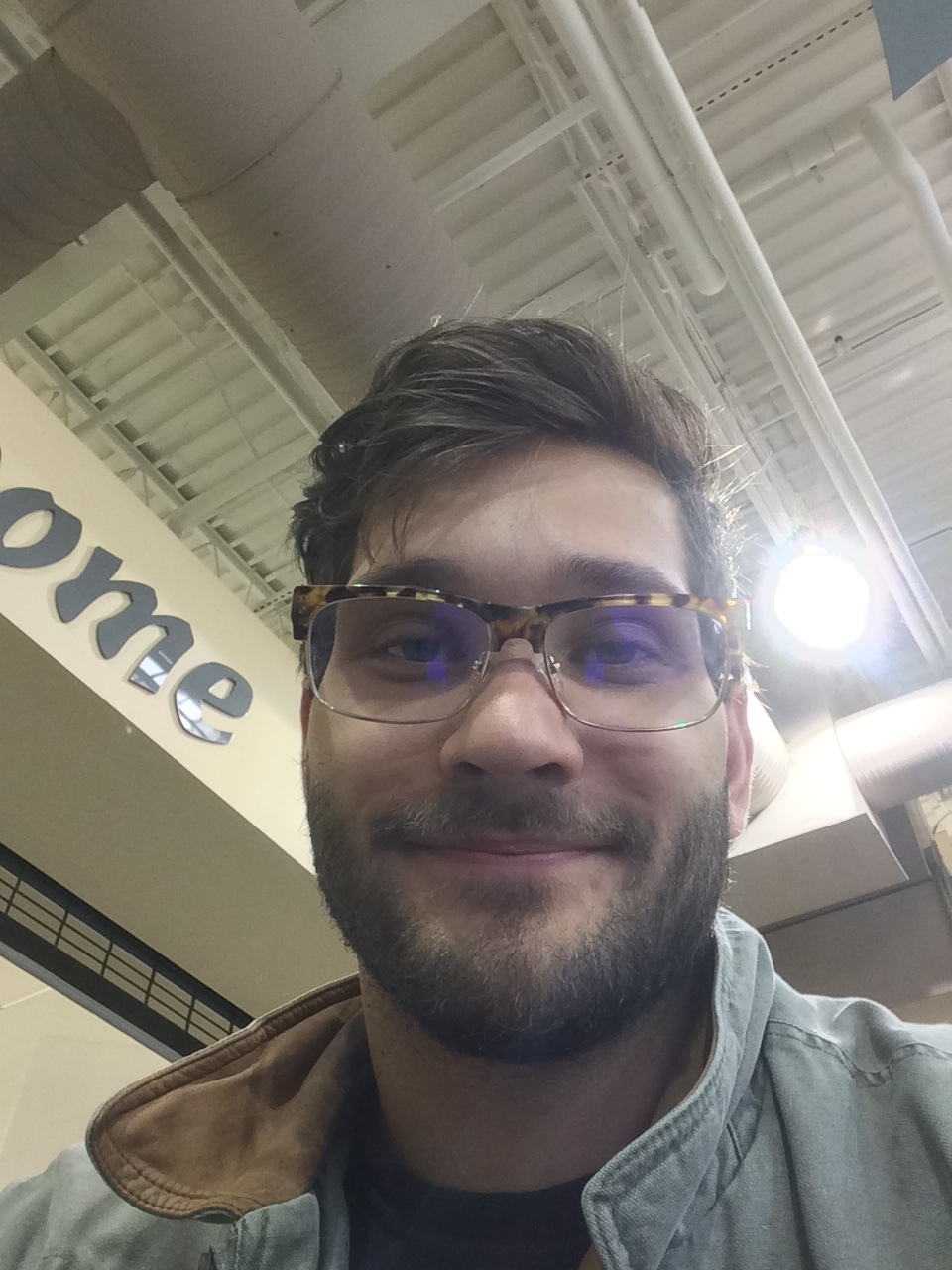My name is Thomas Chuna. I go by TC.

Life has brought me through many different research fields. In college I earned a dual bachelors in math and physics. In math, I studied matrix representations of mapping class groups (thesis here). In physics,  I studied nuclear experimentation. I contributed to a high precision beta decay experiment at Michigan State’s National Superconducting Cyclotron (NSCL). To prepare for that experiment, I simulated radiation transport inside the detector apparatus using Wittenberg university’s computing cluster (APS-DNP abstract). Then, in 2016, I traveled to the NSCL to conduct the experiment (APS-DNP abstract). During my Master’s, I held a research position in finite temperature lattice quantum chromodynamics (LQCD). Using Michigan State’s high performance computing cluster ICER, I developed anisotropic lattices for MILC (code). In conjunction with these Markov Chain Monte Carlo (MCMC) simulations of SU(3) gauge fields, I also developed anisotropic Lie group integrators for MILC (code). Finally, using autocorrelated time series analysis techniques, I developed efficient Lie group RK integrators for renormalizing / scale setting in finite temperature lattices (paper).

In 2021, I entered a research position with the Murillo Lab! Here I have been studying a host of topics

Ongoing Research:

1. Hybrid Models! At its simplest, a hybrid model is a one which stitches together 2 separate models (model A and B) and runs them simultaneously. Consider a kinetic tritium-hydrogen (TH) gas surrounded by an equilibrated carbon-deuterium (CD) foam. A hybrid model can simulate the evolution of the system using a kinetic model in the TH gaseous pore and using a hydrodynamic model in the CD foam. What’s the advantage of a hybrid model? Well, kinetic models provide more degrees of freedom (DoF) than a hydrodynamics model, but the additional DoF raise computational cost. So using the hydrodynamics model where the additional DoF aren’t necessary can save computational cost and preserve model fidelity. (APS-DPP 2022 abstractpaper)
2. Linear Response Theory! Dynamical systems are often intractable to analytic tools. However, if we linearize the system’s description then many analytic tools can now be used to study the system. Linear response theory allows use to determine many physical properties of a system (e.g. susceptibility, conductivity, compressibility).  Recently published multispecies kinetic models have been analyzed using linear response theory. (MIPSE 2022 abstract, upcoming ICOPS 2023 abstract, paper in preparation)
3. Machine Learning Model Closures! In the continuity equation, the dynamics of the density (0th moment) are determined by the divergence of the current density (1st moment). In general, the dynamics of the nth moment require the (n+1)th moment. This collection of n equations has n+1 unknowns. To solve the system of equations, the n+1 moment must be rewritten in terms of the previous n variables. This is constraint is called a model closure. Recently there has been interest in having neural nets predict the n+1 moment using the other n moments (i.e. use a NN closure). This allows optimization techniques to determine the form of the closure.

Education:

• BSc, Physics and Mathematics with Computational Science and Computer Science minors, 2012 – 2016
Wittenberg University, United States
• MSc, Physics, 2016 – 2020
Michigan State University, United States
• PhD, Physics and Computational Mathematics, Science and Engineering, 2020 – Present
Michigan State University, United States

Publications:

Contact:

chunatho@msu.edu